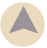# Percentage Calculator

Calculate the value resulting from taking a percentage of a number. For example, calculate what 25 percent of 1500 is (answer: 375), or what 17% of 5000 is (answer: 850). This basic percentage calculation is useful in everyday tasks, including calculating savings when a percent discount applies to a product, calculating sales tax, and calculating ingredient amount in a glaze recipe where the formula is based on percentages.

## Calculate the percentage of a number

percent of
Equals?

### More Percentage Calculators